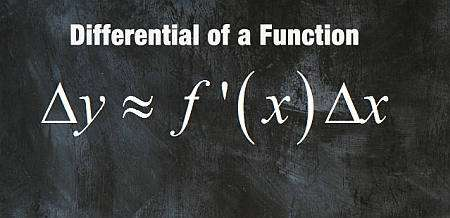# Difference Quotient Calculator

Instructions: Use this calculator to find the difference quotient of any function that you provide, showing all the steps. Please use the form below to type a valid function:Enter the function for which you want to calculate the difference quotient (Ex: f(x) = 3x^2 + 1, etc.)

## Difference Quotient Calculator

This calculator will allow you to compute a difference quotient for any valid function you provide, showing all the steps. Make sure you provide a valid function that does not lead to any ambiguities, placing parentheses in the correct places, to avoid unintended calculations.

For example, f(x) = sin 2 x - 2 is ambiguous, cause you could be meaning sin(2) * x -2, or sin(2x)-2 or sin(2x-2), which are all different. So it depends on where you place the parenthesis. If you put no parentheses, the system will interpret f(x) = sin 2 x - 2 as f(x) = (sin(2))*x - 2, which likely not what was intended.

Then, when a valid function is provided, you need to click on "Calculate" to get all the steps shown of the difference quotient calculations.

Difference quotients are very important because they are tightly linked to the calculation of derivatives, and they have a geometric interpretation of being the slope of a secant line, as well as an average rate of change.## Difference Quotient Formula

The difference quotient is something you compute for a given function $$f(x)$$. The difference quotient formula is

$\displaystyle \frac{f(x+h)-f(x)}{h}$

Does this look similar to something you know? Of course, it looks like the derivative formula, only without the limit. So, when computing derivatives you are actually first computing a difference quotient and then you are taking a limit has $$h$$ approaches to 0.

## Steps for calculating difference quotients

• Step 1: Identify clearly the function f(x) you want to work with. Make sure the function is validly defined before moving forward
• Step 2: Once you know that f(x) is valid, you evaluate the function at two generic values x + h and x, and compute the difference f(x+h) - f(x)
• Step 3: Then divide what you found above by h, so you get (f(x+h)-f(x))/h, which is the difference quotient
• Step 4: Simplify the expression you found above as much as possible

The difference quotient is usually worked out in terms of the calculation of the derivative, but not always, as often times you are going to use it a the average rate of change of a function, when the value of the argument changes from x to x+h.

## Using a difference quotient calculator

This difference quotient calculator will show you step by step what is needed to get to the final result, from the setting of the term of the quotient to the simplification of the final expression.

Observe that there is an alternative form, which is

$\displaystyle \frac{f(x)-f(a)}{x-a}$

but naturally you see if you define $$h = x-a$$ you have $$x = a+h$$ and you land into the original form.## Why would you need difference quotients?

As we were talking about in the previous section, difference quotients are essentially the preamble calculation needed to differentiate functions. So then they do play a very important role.

Also, the ability to get the simplified difference quotient will make it possible to find the limit that defines a derivative, whenever the basic derivative rules don't apply and we are forced to compute the derivative by hand.### Example: Calculating the difference quotient of a function

Find the difference quotient of: $$f(x) = x^2 + 2x - 4$$

Solution:

which concludes the calculation.

### Example: Difference quotient of the square root

Find the difference quotient of : $$f(x) = \sqrt x$$

Solution: By simply plugging the values of $$x+h$$ and $$x$$ in the function, we get

$\displaystyle \frac{f(x+h)-f(x)}{h} = \displaystyle \frac{\sqrt{x+h}-\sqrt x}{h}$

Rationalizing:

$\displaystyle \frac{(\sqrt{x+h}-\sqrt x)(\sqrt{x+h}+\sqrt x)}{h(\sqrt{x+h}+\sqrt x)}$ $= \displaystyle \frac{x+h-x}{h(\sqrt{x+h}+\sqrt x)}$ $= \displaystyle \frac{h}{h(\sqrt{x+h}+\sqrt x)}$

which concludes the calculation.

## More Calculus solvers

One of the most useful tools you will find for Calculus is a derivative calculator, which will compute a derivative for you showing all the steps. Most everything you do in Calculus stems from the calculation of derivatives.

Tightly related to difference quotient, you have the idea of tangent line, which reflects some kind of instantaneous difference quotient.